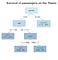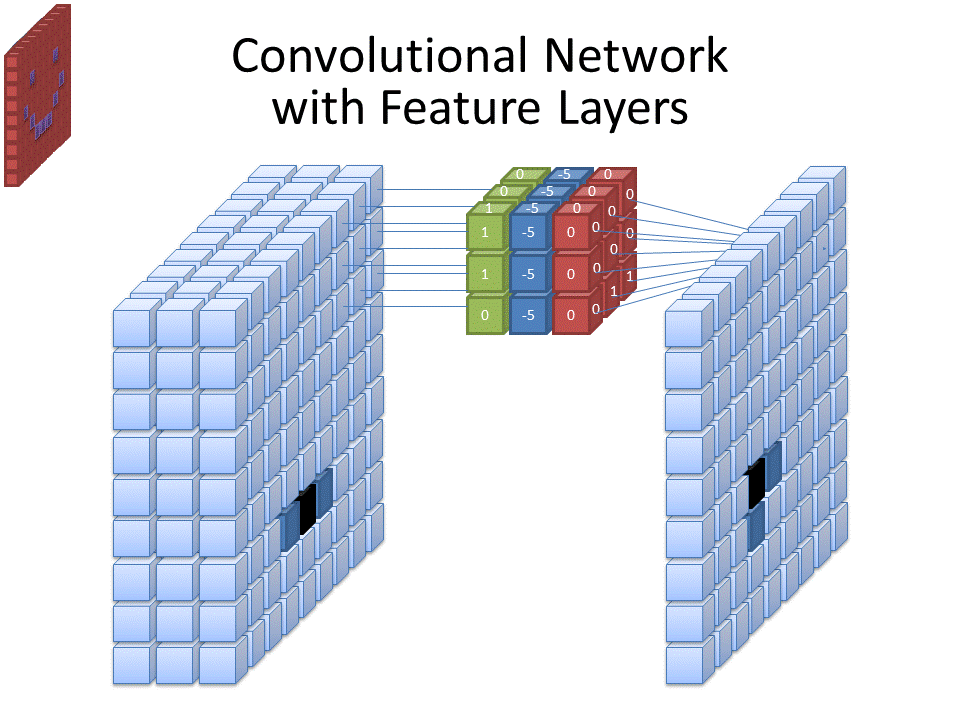# Logistic Regression: Machine Learning

Logistic regression is a statistical model that performs predictions for categorical variables for instance “success” or “failure”, “yes” or “no”, “win” or “loss”. In most cases, logistic regression is used to solve the binary classification problem. But we can also use logistic regression in multi-class classification.

# Logistic Function

The logistic function is an “S” shape curve that maps predicted values to probabilities. Target variable y is the probability of a given variable x belonging to a certain class. Because the probability lies from the range 0 to 1 Thus, it is obvious that the value of y should lie between 0 and…

# Introduction of Decision tree

A decision tree is a classification algorithm that comes under the supervised learning technique. This algorithm is very easy to understand and explain. There are very few algorithms that fall into this category. A decision tree is a graphical representation of all the possible solutions to a decision based on certain conditions. Perhaps you are wondering why the machine learning community came up with the name decision tree. Well, if you look carefully at the figure of the algorithm, you will see it as a tree.Decision tree

Decision trees mimic the human brain. The human brain splits up any task into…

# Principal Component Analysis

The principal component analysis is an unsupervised learning technique to overcome the problem of high dimensionality in the training dataset. The primary problem associated with high-dimensionality in the machine learning field in model overfitting, which reduces the ability to generalize beyond the examples in the training set. It is also known as the curse of dimensionality. The main goal of PCA is to convert data from high-dimensional space to low-dimensional space. Another problem with high dimensions is that it always affects the accuracy of the model. …

# AlexNet CNN Network

AlexNet was a groundbreaking development in the field of modern convolutional neural networks. It was developed by Alex Krizhevsky in collaboration with Geoffrey Hinton. The network won the 2012 ImageNet challenge 10.8% lower error rate than the runner up model. It got a 15.3% top five error rate in the competition. This network has 60 million parameters, and it was not possible to train such a large network without high-speed computing power. Therefore, in the training phase, the neurons of the network were split half-half into two GTX-580 GPUs.

The ImageNet dataset contains 1.2 million training images, 50,000 validation images

# LeNet-5: the foundation stone of Convolutional Neural NetworksConvolutional Neural network

Computer vision is an artificial neural network in which a computer can learn to recognize images and perform object detection. In the 1950s, extensive research on artificial neural networks began. In 1959, David Hubble and Torsten Wiesel pointed out that the human visual cortex is primarily composed of simple cells and complex cells. The simple cell responds to the edges of the orientation, on the other hand, the complex cell is different from the simple cell in the sense that even if we shift the bars and edges of the image, the complex cell still responds.

In 1980, Kunihiko Fukushima

# Machine Learning: Linear Regression

Linear regression is a supervised learning technique. Which demonstrates the relationship between a dependent variable and one or more independent variables? It works under the condition that the dependent variable is continuous or real (such as monthly salary data).

# Simple Linear Regression

Simple linear regression is a method of modeling the relationship between the dependent variable y and a single independent variable x.